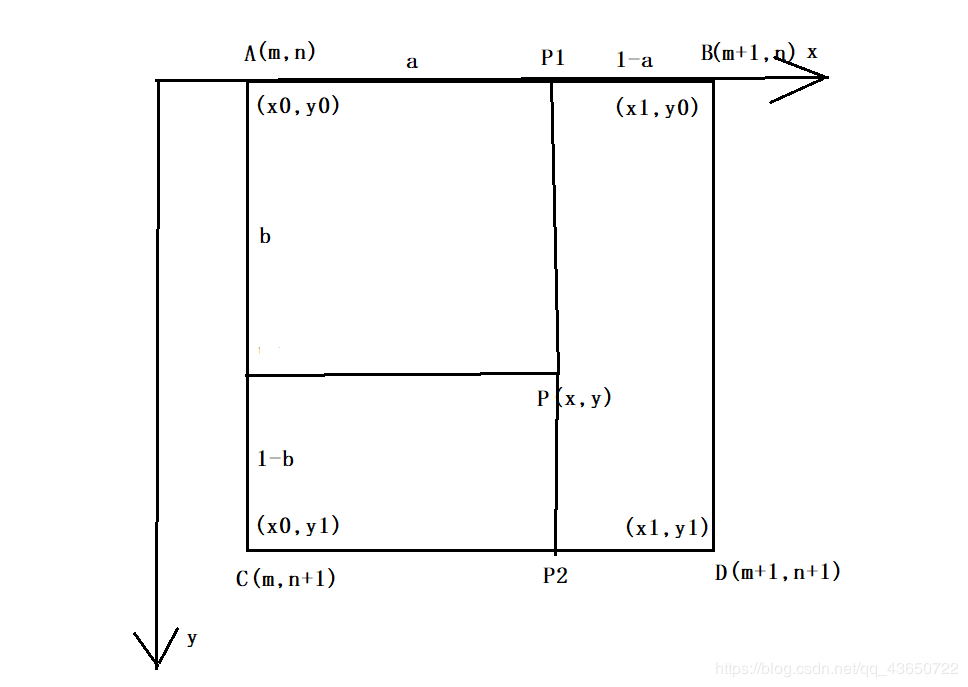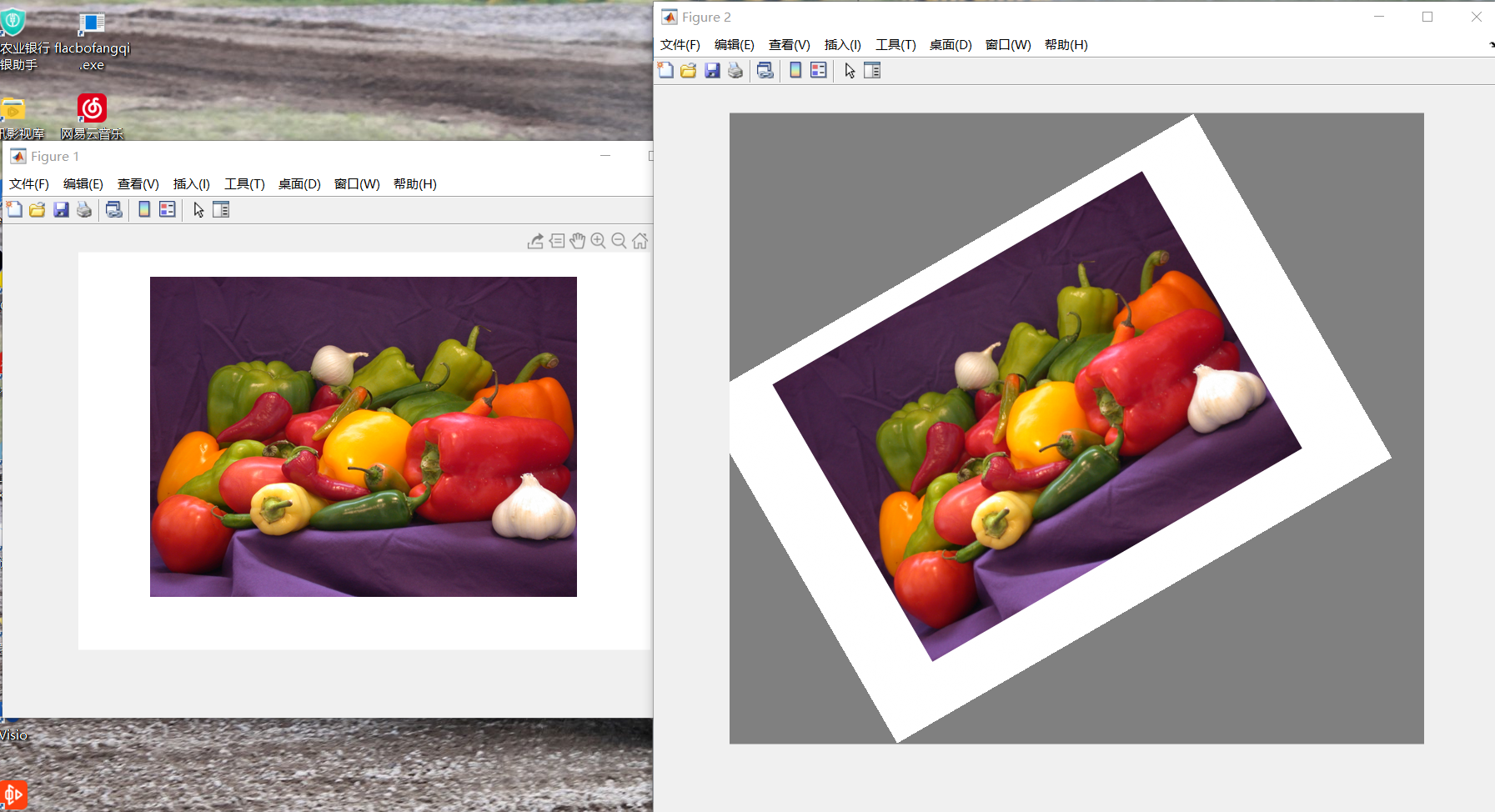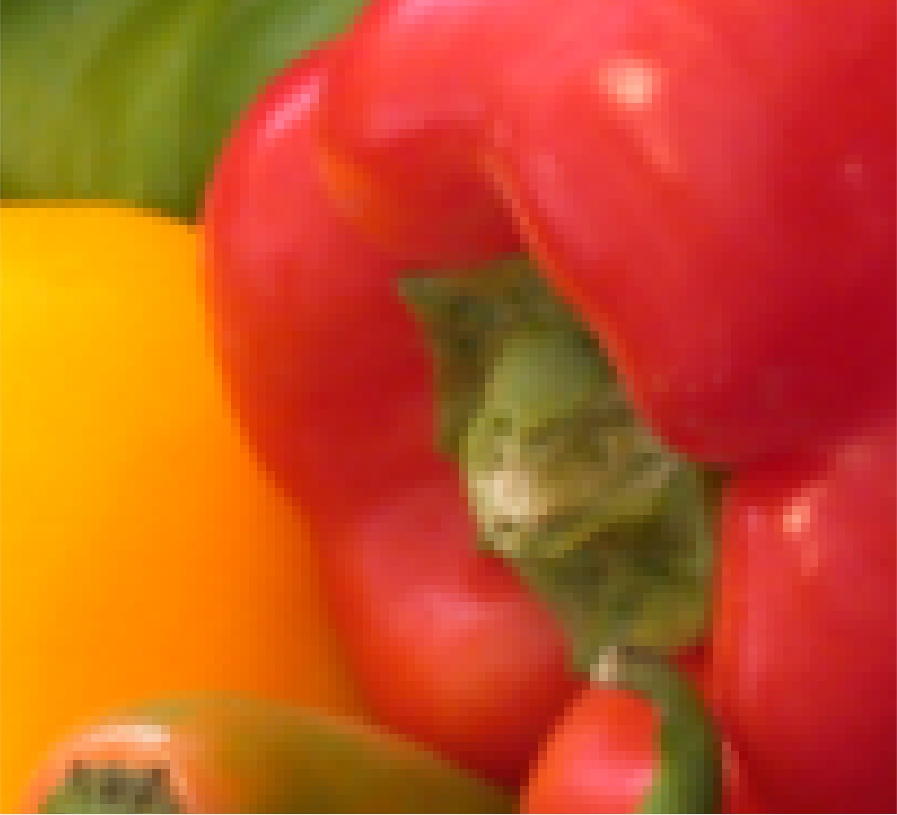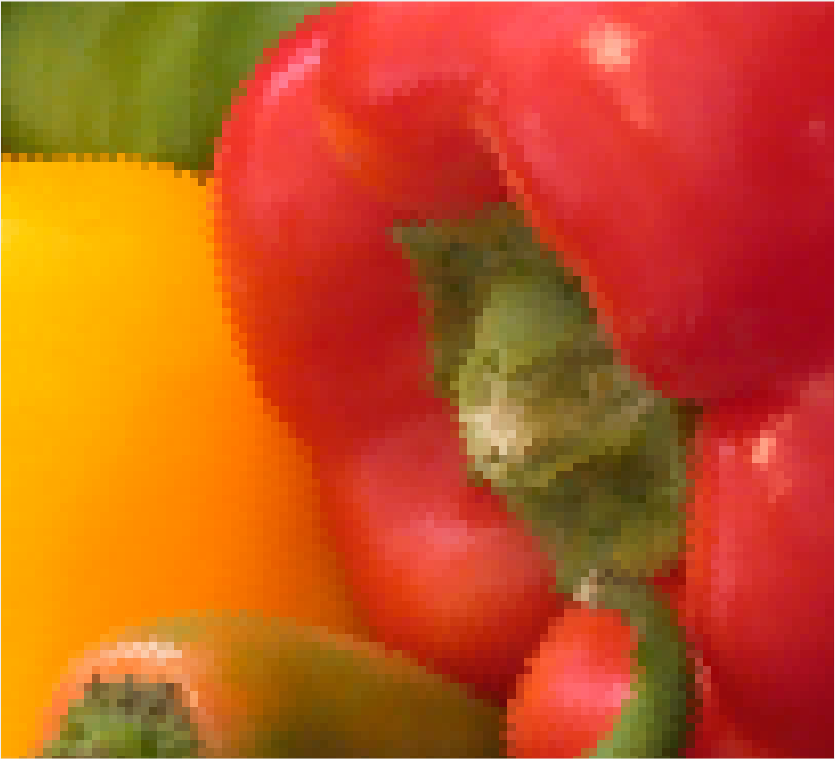# 二、本设计中的改善

im=imread('fruit.png');

%旋转30°
%求出旋转矩阵
a=30/180*pi;
R=[cos(a),-sin(a);sin(a),cos(a)];%旋转矩阵
R=R';%求出旋转矩阵的逆矩阵

%求出图片大小
sz=size(im);
h=sz(1);
w=sz(2);
ch=sz(3);
c1=[h;w]/2;%原图中心

%计算显示完整图像需要的画布大小
hh=floor(width*sin(a)+height*cos(a))+1;
ww=floor(width*cos(a)+height*sin(a))+1;
c2=[hh,ww]/2;%求新画布中点

%初始化目标画布
im2=uint8(ones(hh,ww,3)*128);
for k = 1:ch
for i = 1:hh
for j = 1:ww
p=[i;j];%遍历新图像像素点
pp=round(R*(p-c2)+c1);%计算在原来图像中的位置
%这里仍然使用round函数，但结果会比方案一好得多
%逆向进行像素查找
if(pp(1)>=1&&pp(1)<=h&&pp(2)>=1&&pp(2)<=w)
im2(i,j,k)=im(pp(1),pp(2),k);
end
end
end
end

%显示图像
figure;
imshow(im);
figure;
imshow(im2);



im=imread('fruit.png');

%旋转30°
%求出旋转矩阵
a=30/180*pi;
R=[cos(a),-sin(a);sin(a),cos(a)];%旋转矩阵
R=R';%求出旋转矩阵的逆矩阵

%求出图片大小
sz=size(im);
h=sz(1);
w=sz(2);
ch=sz(3);
c1=[h;w]/2;%原图中心

%计算显示完整图像需要的画布大小
hh=floor(width*sin(a)+height*cos(a))+1;
ww=floor(width*cos(a)+height*sin(a))+1;
c2=[hh,ww]/2;

%初始化目标画布
im2=uint8(ones(hh,ww,3)*128);
for k = 1:ch
for i = 1:hh
for j = 1:ww
p=[i;j];%后图像
pp=(R*(p-c2)+c1);%计算在原来图像中的位置
mn=floor(pp);
ab=pp-mn;%这里就代表了该坐标点离左上点的位置
a=ab(1);
b=ab(2);
m=mn(1);
n=mn(2);
%线性差值方法
if(pp(1)>=1&&pp(1)<=h&&pp(2)>=1&&pp(2)<=w)
im2(i,j,k)=(1-a)*(1-b)*im(m,n,k)+a*(1-b)*im(m+1,n,k)...
+(1-a)*b*im(m,n+1,k)+a*b*im(m+1,n+1,k);
end
end
end
end

%显示图像
figure;
imshow(im);
figure;
imshow(im2);


pp=(R*(p-c2)+c1);%计算在原来图像中的位置
mn=floor(pp);
ab=pp-mn;%这里就代表了该坐标点离左上点的位置
a=ab(1);
b=ab(2);
m=mn(1);
n=mn(2);
%线性差值方法
if(pp(1)>=1&&pp(1)<=h&&pp(2)>=1&&pp(2)<=w)
im2(i,j,k)=(1-a)*(1-b)*im(m,n,k)+a*(1-b)*im(m+1,n,k)...
+(1-a)*b*im(m,n+1,k)+a*b*im(m+1,n+1,k);
endP = A ( 1 − a ) ( 1 − b ) + B ∗ a ( 1 − b ) + C ( 1 − a ) b + D ∗ a ∗ b ; P=A(1-a)(1-b)+B*a(1-b)+C(1-a)b+D*a*b;
MATLAB代码表示就是:

im2(i,j,k)=(1-a)*(1-b)*im(m,n,k)+a*(1-b)*im(m+1,n,k)...
+(1-a)*b*im(m,n+1,k)+a*b*im(m+1,n+1,k);03-103054
06-29453
11-221183
03-18991
12-086477
06-282985